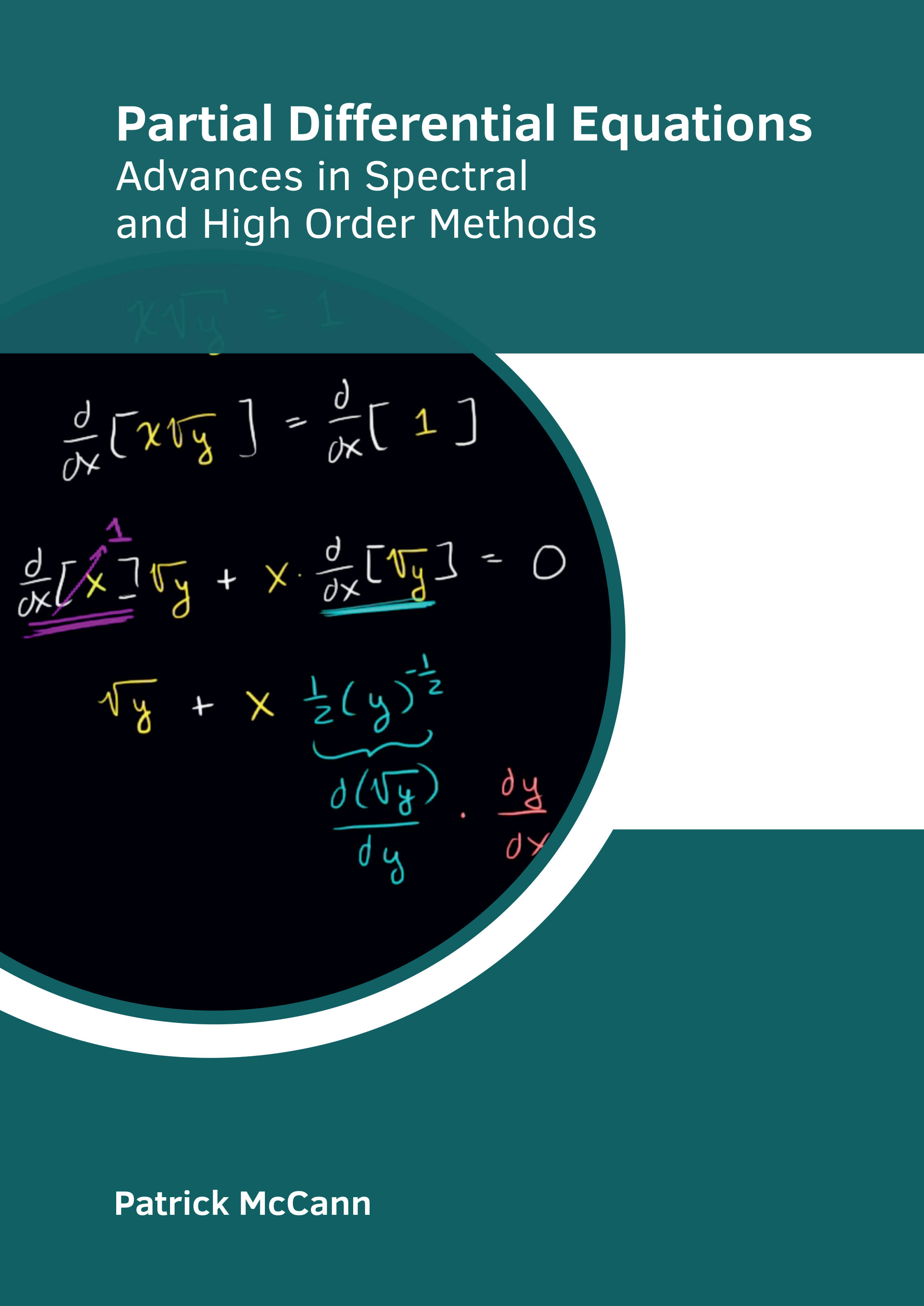# Books /Partial Differential Equations: Advances in Spectral and High Order Methods

BROWSE BY

### Partial Differential Equations: Advances in Spectral and High Order Methods9781639894048

Author

Patrick McCann

English

##### Publication Year

2022

Category :

Mathematics and Statistics - Differential Equations

\$ 157.95

##### Description

Partial differential equations (PDEs) are mathematical equations that relate a function of several variables to its partial derivatives. A partial derivative of a function of many variables describes how fast the function changes when one variable is altered, keeping the others constant. The task of discovering a partial derivative can be applied to a function that is itself a partial derivative of another function to obtain a second-order partial derivative. The order and degree of partial differential equations are determined in the same way as ordinary differential equations. Different approaches, evaluations and methodologies on partial differential equations have been included in this book. It strives to provide a fair idea about this discipline and to help develop a better understanding of the latest advances within this field. Coherent flow of topics, student-friendly language and extensive use of examples make this book an invaluable source of knowledge.